# How to convert text to number in Excel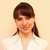by , updated on

The tutorial shows many different ways to turn a string into a number in Excel: Convert to Number error checking option, formulas, mathematic operations, Paste Special, and more.

Sometimes values in your Excel worksheets look like numbers, but they don't add up, don't multiply and produce errors in formulas. A common reason for this is numbers formatted as text. In many cases Microsoft Excel is smart enough to convert numerical strings imported from other programs to numbers automatically. But sometimes numbers are left formatted as text causing multiple issues in your spreadsheets. This tutorial will teach you how to convert strings to "true" numbers.

## How to identify numbers formatted as text in Excel

Excel has an inbuilt error checking feature that alerts you about possible problems with cell values. This appears as a small green triangle in the top left corner of a cell. Selecting a cell with an error indicator displays a caution sign with the yellow exclamation point (please see the screenshot below). Put the mouse pointer over the sign, and Excel will inform you about the potential issue: The number in this cell is formatted as text or preceded by an apostrophe.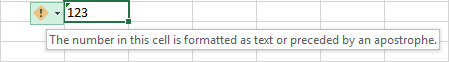In some cases, an error indicator does not show up for numbers formatted as text. But there are other visual indicators of text-numbers:

 Numbers Strings (text values) Right-aligned by default. If several cells are selected, the Status Bar shows Average, Count and SUM. Left-aligned by default. If several cells are selected, the Status Bar only shows Count. The Number Format box displays the Text format (in many cases, but not always). There may be a leading apostrophe visible in the formula bar.

In the image below, you can see the text representations of numbers on the right and actual numbers on the left: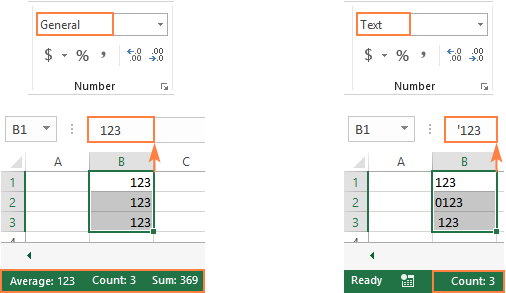## How to convert text to number in Excel

There are a handful of different ways to change text to number of Excel. Below we will cover them all beginning with the fastest and easiest ones. If the easy techniques don't work for you, please don't get disheartened. There is no challenge that cannot be overcome. You will just have to try other ways.

### Convert to number in Excel with error checking

If your cells display an error indicator (green triangle in the top left corner), converting text strings to numbers is a two-click thing:

1. Select all the cells containing numbers formatted as text.
2. Click the warning sign and select Convert to Number.

Done!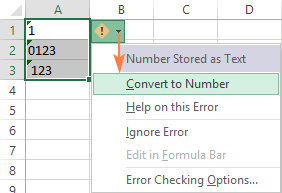### Convert text into number by changing the cell format

Another quick way to convert numerical values formatted as text to numbers is this:

1. Select the cells with text-formatted numbers.
2. On the Home tab, in the Number group, choose General or Number from the Number Format drop-down list.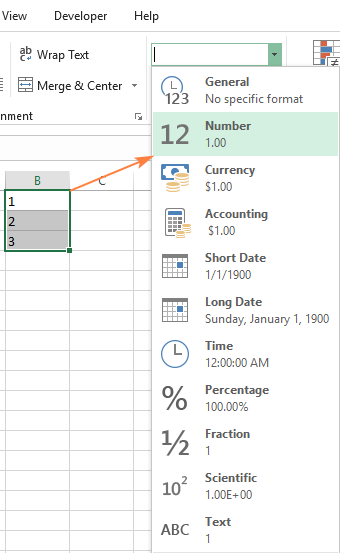Note. This method does not work in some scenarios. For example, if you apply the Text format to a cell, enter a number, and then change the cell format to Number, the cell will remain formatted as text.

### Change text to number with Paste Special

Compared to the previous techniques, this method of converting text to number requires a few more steps, but works almost 100% of time.

To fix numbers formatted as text with Paste Special, here's what you do:

1. Select the text-number cells and set their format to General as explained above.
2. Copy a blank cell. For this, either select a cell and press Ctrl + C or right-click and choose Copy from the context menu.
3. Select the cells you want to convert to numbers, right-click, and then click Paste Special. Alternatively, press the Ctrl + Alt + V shortcut.
4. In the Paste Special dialog box, select Values in the Paste section and Add in the Operation section.
5. Click OK.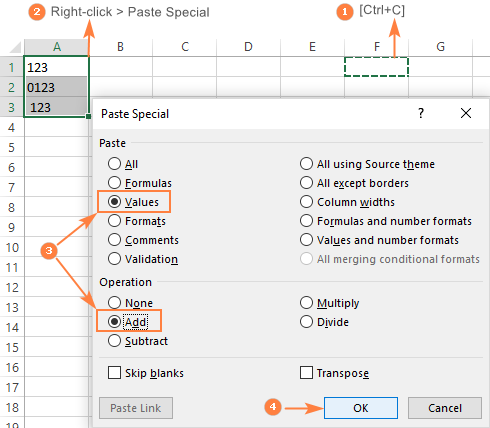If done correctly, your values will change the default alignment from left to right, meaning Excel now perceives them as numbers.

### Convert string to number with Text to Columns

It is another formula-free way to convert text to number in Excel. When used for other purposes, for example to split cells, the Text to Columns wizard is a multi-step process. To perform the text to number conversion, you click the Finish button in the very first step :)

1. Select the cells you'd like to convert to numbers, and make sure their format is set to General.
2. Switch to the Data tab, Data Tools group, and click the Text to Columns button.
3. In step 1 of the Convert Text to Columns Wizard, select Delimited under Original data type, and click Finish.

That's all there is to it!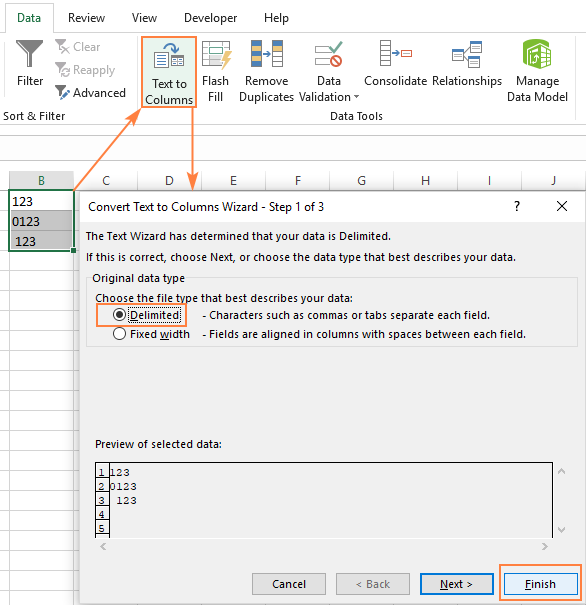### Convert text to number with a formula

So far, we have discussed the built-in features that can be used to change text to number in Excel. In many situations, a conversion can be done even faster by using a formula.

#### Formula 1. Convert string to number in Excel

Microsoft Excel has a special function to convert a string to number - the VALUE function. The function accepts both a text string enclosed in quotation marks and a reference to a cell containing the text to be converted.

The VALUE function can even recognize a number surrounded by some "extra" characters - it's what none of the previous methods can do.

For example, a VALUE formula recognizes a number typed with a currency symbol and a thousand separator:

`=VALUE("\$1,000")`

`=VALUE(A2)`

To convert a column of text values, you enter the formula in the first cell, and drag the fill handle to copy the formula down the column: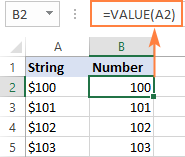#### Formula 2. Convert string to date

Apart from text-numbers, the VALUE function can also convert dates represented by text strings.

For example:

`=VALUE("1-Jan-2018")`

Or

`=VALUE(A2)`

Where A2 contains a text-date.

By default, a VALUE formula returns a serial number representing the date in the internal Excel system. For the result to appear as an actual date, you just have to apply the Date format to the formula cell.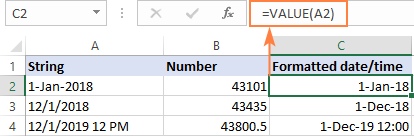The same result can be achieved by using the DATEVALUE function:

`=DATEVALUE(A2)`

#### Formula 3. Extract number from string

The VALUE function also comes in handy when you extract a number from a text string by using one of the Text functions such as LEFT, RIGHT and MID.

For example, to get the last 3 characters from a text string in A2 and return the result as a number, use this formula:

`=VALUE(RIGHT(A2,3))`

The screenshot below shows our convert text to number formula in action: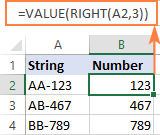If you don't wrap the RIGHT function into VALUE, the result will be returned as text, more precisely a numeric string, which makes any calculations with the extracted values impossible.

### Change Excel string to number with mathematic operations

One more easy way to convert a text value to number in Excel is to perform a simple arithmetic operation that does not actually change the original value. What can that be? For example, adding a zero, multiplying or dividing by 1.

`=A2+0`

`=A2*1`

`=A2/1`

If the original values are formatted as text, Excel may automatically apply the Text format to the results too. You may notice that by the left-aligned numbers in the formula cells. To fix this, be sure to set the General format for the formula cells.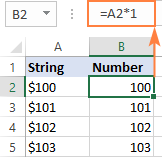Tip. If you'd like to have the results as values, not formulas, use the Paste Special feature to replace formulas with their values.

That's how you convert text to number in Excel with formulas and built-in features. I thank you for reading and hope to see you on our blog next week!